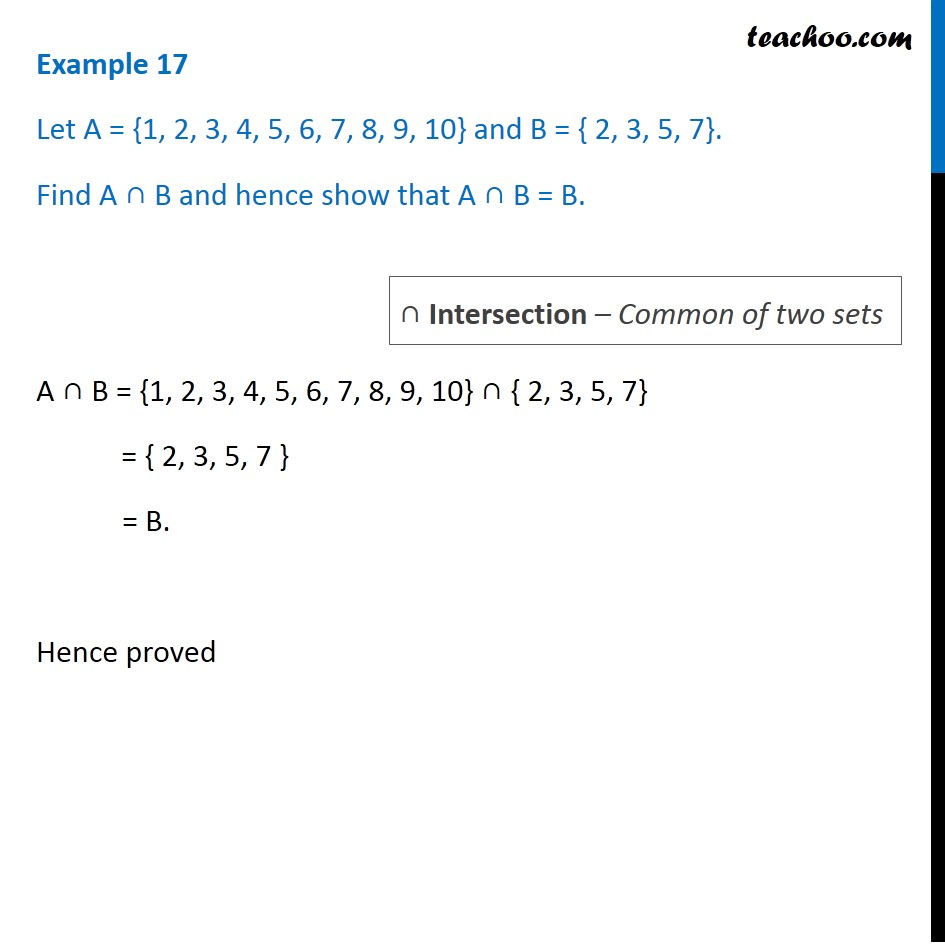1. Chapter 1 Class 11 Sets (Term 1)
2. Serial order wise
3. Examples

Transcript

Example 17 Let A = {1, 2, 3, 4, 5, 6, 7, 8, 9, 10} and B = { 2, 3, 5, 7}. Find A ∩ B and hence show that A ∩ B = B. A ∩ B = {1, 2, 3, 4, 5, 6, 7, 8, 9, 10} ∩ { 2, 3, 5, 7} = { 2, 3, 5, 7 } = B. Hence proved ∩ Intersection – Common of two sets

Examples

Chapter 1 Class 11 Sets (Term 1)
Serial order wise